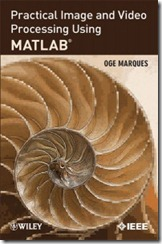## Tuesday, October 4, 2011

### “Practical Image and Video Processing Using MATLAB®”This is the first book to combine image and video processing with a practical MATLAB(R)-oriented approach in order to demonstrate the most important image and video techniques and algorithms. Utilizing minimal math, the contents are presented in a clear, objective manner, emphasizing and encouraging experimentation.

The book has been organized into two parts. Part I: Image Processing begins with an overview of the field, then introduces the fundamental concepts, notation, and terminology associated with image representation and basic image processing operations. Next, it discusses MATLAB(R) and its Image Processing Toolbox with the start of a series of chapters with hands-on activities and step-by-step tutorials. These chapters cover image acquisition and digitization; arithmetic, logic, and geometric operations; point-based, histogram-based, and neighborhood-based image enhancement techniques; the Fourier Transform and relevant frequency-domain image filtering techniques; image restoration; mathematical morphology; edge detection techniques; image segmentation; image compression and coding; and feature extraction and representation.

Part II: Video Processing presents the main concepts and terminology associated with analog video signals and systems, as well as digital video formats and standards. It then describes the technically involved problem of standards conversion, discusses motion estimation and compensation techniques, shows how video sequences can be filtered, and concludes with an example of a solution to object detection and tracking in video sequences using MATLAB(R).

Extra features of this book include:

More than 30 MATLAB(R) tutorials, which consist of step-by-step guides to exploring image and video processing techniques using MATLAB(R)

Chapters supported by figures, examples, illustrative problems, and exercises

Useful websites and an extensive list of bibliographical references

This accessible text is ideal for upper-level undergraduate and graduate students in digital image and video processing courses, as well as for engineers, researchers, software developers, practitioners, and anyone who wishes to learn about these increasingly popular topics on their own.

http://www.ogemarques.com/